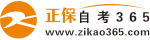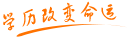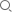#24小时客服：010-82335555# 自考“C语言程序设计”模拟试题八答案

2007年05月29日    来源：   字体：   打印

一、选择题

1.D       2.  C        3. A         4.  C       5.  B

二、填空题

1.字母、数字、下划线；以字母、下划线开头。

2.10；5.

3. 该变量的地址。

4.一组数据类型相同的元素构成的；一组数据类型不同的元素构成的。

5.  打开；关闭。

6.字符串长度、取子字符串、字符串替换、字符串复制等。

7. 函数；语句。

8. int a ；  float b ；  char c ；

9   9   9.3   6   1   1  1   2

三、判断题

1. 对   2.  错    3. 错   4. 错  5. 对  6. 错  7. 对  8. 错  9.对  10. 错

四、程序填空题

1.   i<=9     j<=9

printf（“%d*%d=%d”，i，j，i*j）；

printf（“\n”）； i ， j ， k；

2.  int z；

if （x>=y）   z=x；

else          z=y；

return  z；

3.  char  name；

char  sex；

int  age；

float  score；

4. 实现功能：计算连续数之积，  即sum=1*2*3*4*5  ；

运行结果：sum=120

5. 44   22   946

a++：先加1，后使用表达式的值；++a表示先加1，后使用表达式的值，它们最后的结果使a加1.

6.45，15

五、编程题

1.编程实现对12个整数进行冒泡排序（要求排成升序）。

Main（）

{   int  a；  int  m，n，p；

for （m=0；m<=11；m++）（m=0；m<=11；m++）

scanf（“%d”，&a[m]）；

for （m=0；m<=11；m++）

for（n=0；n<11-m；n++）

if（a[n]>a[n+1]）

{   p=a[n]；   a[n]=a[n+1]；    a[n+1]=p；   }

for （m=0；m<=11；m++）

printf（“%d”，a[m]）；                  }

2.编程实现求下面的式子的结果

# include “math.h”

main（）

{  float x，y；

scanf（“%f”&x）；

if （x>6）   y=（x-3）/（2*x-12）；

else       y=-2*x*x+4*x-10；

printf（“y=%f”，y）；                       }

3.编程实现将文本文件file1.dat中的内容复制到文本文件file2.dat中去

main（）

{  char ch；

FILE *fp1，*fp2；

Fp1=fopen（“file1.dat”， “r”）；

Fp2=fopen（“file2.dat”， “w”）；

Ch=fgetc（fp1）；

While（ch！=EOF）

{   fputc（ch，fp2）；

Ch=fgetc（fp1）；  }

Fclose（fp1）；

Fclose（fp2）；                 }

六、附加题

p=H；K=0；

While（p！=NULL && k<i-1）

{   p=p->next；

k++；           }

if（p==NULL  &&  H！=NULL）   printf（“this is invilid”）；

else

{  q=（Struct Linklist *）malloc（sizeof（Struct Linklist））；

q->data=x； q->data=x；

q->next=p->next；

p->next=q；            }

• 热门专业
• 报名咨询

• 最新问答
• 热门问答